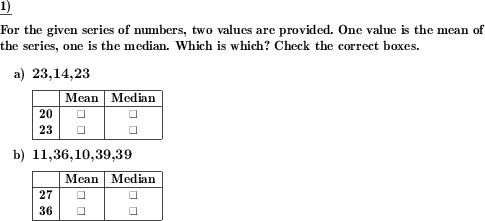Custom math worksheets at your fingertips# Details for problem "Median and mean - decide which number is which"

Quickname: 9556

Elementary School, Primary School, Junior High School, Middle School, High School.

## Summary

For two given numbers for a number series, decide which is the median and which is the mean.

## Example## Description

A series of whole numbers is presented. In addition, two more numbers of integers are presented. One of these numbers is the mean, one number is the median. The task is to identify which number is which.

The number space from which the numbers come can be set. The number of numbers in the number series can also be selected. If the median is asked for, it must be calculated from the mean value of the two middle numbers if the number of numbers is even. To ensure that the median can always be read directly from the number series, it can be specified that the number of numbers is always odd when the median is asked for.

The number series is designed in such a way that the mean and the median, like the numbers themselves, are always whole numbers.

If desired, the numbers can be presented sorted by size.

Download free printable worksheets for this math problem here. The worksheet contains the problems only, the solution sheet includes the answers. Just click on the respective link.

•Worksheet 1Solution sheet with answers
•Worksheet 2Solution sheet with answers
•Worksheet 3Solution sheet with answers

If you can not see the solution sheets for download, they may be filtered out by an ad blocker that you may have installed. If this is the case, please allow ads for this page and reload the page. The solution sheets will then reappear.

• Do these sample worksheets do not really fit?
• Do you need more math worksheets, with a different level of difficulty?
• Would you like to combine different problems on a worksheet and adjust them to your needs?
• As a teacher, you can put together your own worksheets using the automatically generated math problems provided.
With a free initial credit, you can start creating your own math worksheets in a few minutes.

You can try it for free! Register here, to create custom worksheets now!

## Customization options for this problem

Parameter
Possible values
Number of problems
1, 2, 3, 4, 5
Number of numbers
3-7, 7-9, 9-11
Even number of numbers allowed
Yes, No
Same number allowed multiple times
Yes, No
Sorted list of numbers given
Yes, No
Number range
1 - 50, 1 - 100, -20 - 20, -50 - 50, -100 - 100

## Similar problems

Remark
Description
Problem without given values for median or mean value
For a series of numbers, the mean or median is to be found.
For a given value, decide whether it is the median or the mean value
Decide whether a number is the median or mean of a given series of whole numbers.